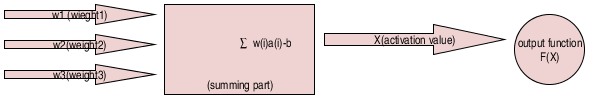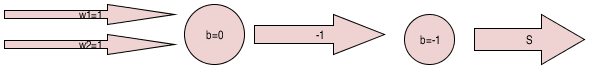# Welcome to the Learning Concepts of Neural Networks MCQs Page

Dive deep into the fascinating world of Learning Concepts of Neural Networks with our comprehensive set of Multiple-Choice Questions (MCQs). This page is dedicated to exploring the fundamental concepts and intricacies of Learning Concepts of Neural Networks, a crucial aspect of Neural Networks. In this section, you will encounter a diverse range of MCQs that cover various aspects of Learning Concepts of Neural Networks, from the basic principles to advanced topics. Each question is thoughtfully crafted to challenge your knowledge and deepen your understanding of this critical subcategory within Neural Networks.

Check out the MCQs below to embark on an enriching journey through Learning Concepts of Neural Networks. Test your knowledge, expand your horizons, and solidify your grasp on this vital area of Neural Networks.

Note: Each MCQ comes with multiple answer choices. Select the most appropriate option and test your understanding of Learning Concepts of Neural Networks. You can click on an option to test your knowledge before viewing the solution for a MCQ. Happy learning!

### Learning Concepts of Neural Networks MCQs | Page 1 of 5

Q1.
What is the name of the model in figure below?Q2.
What is nature of function F(x) in the given figure?Q3.
What does the character ‘b’ represents in the below diagram?Q4.
If ‘b’ in the figure below is the bias, then what logic circuit does it represents?Q5.
When both inputs are 1, what will be the output of the below figure?a.

0

b.

1

d.

z

Q6.
When both inputs are different, what will be the output of the below figure?a.

0

b.

1

d.

z

Q7.
Which of the following model has ability to learn?
Q8.
When both inputs are 1, what will be the output of the pitts model nand gate ?

a.

0

b.

1

d.

z